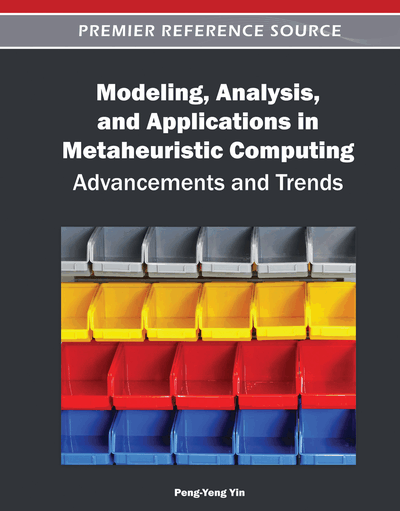# A Study of Tabu Search for Coloring Random 3-Colorable Graphs Around the Phase Transition

Jean-Philippe Hamiez (Université d’Angers/LERIA, France), Jin-Kao Hao (Université d’Angers, France) and Fred W. Glover (OptTek Systems Inc., USA)
Copyright: © 2012 |Pages: 28
DOI: 10.4018/978-1-4666-0270-0.ch012
OnDemand PDF Download:
Available
\$37.50
No Current Special Offers

## Abstract

The authors present an experimental investigation of tabu search (TS) to solve the 3-coloring problem (3-COL). Computational results reveal that a basic TS algorithm is able to find proper 3-colorings for random 3-colorable graphs with up to 11000 vertices and beyond when instances follow the uniform or equipartite well-known models, and up to 1500 vertices for the hardest class of flat graphs. This study also validates and reinforces some existing phase transition thresholds for 3-COL.
Chapter Preview
Top

## Introduction

Given a simple undirected graph G = (V(G), E(G)), where V(G) = {v1, v2, …, vn} is a set of n vertices (n is usually called the “order” of G) and E(G) ⊂ V(G) × V(G) a set of m edges, and a set C = {c1, c2, …, ck} of k colors, a k-coloring of G is any assignment of one of the k available colors from C to every vertex in V(G). More formally, a k-coloring of G is a mapping c: V(G) → C. The k-coloring problem (k-COL) is to find such a mapping (or prove that none exists) such that adjacent vertices receive different colors (called “proper” k-coloring). More formally, a proper k-coloring of G verifies {vi, vj} ∈ E(G) → c(vi) ≠ c(vj). The tightly related optimization version of k-COL is the graph coloring problem (COL): Determine a proper k-coloring of G with k minimum, i.e. the chromatic number χ(G).

k-COL is known to be NP-complete when k ≥ 3 for general graphs (Garey & Johnson, 1979; Karp, 1972). It remains NP-complete even for particular classes of graphs, including, for instance, triangle-free graphs with maximum degree 4 (Maffray & Preissmann, 1996). Classes of graphs for which 3-COL can be decided in polynomial time are discussed, for instance, in (Alekseev et al., 2007; Kochol et al., 2003).

Another way to express the difficulty of a combinatorial search problem is to consider the phase transition phenomenon which refers to the “easy-hard-easy” transition regions where a problem goes from easy to hard, and conversely (Cheeseman et al., 1991; Dubois et al., 2001; Gent et al., 1996; Hartmann & Weigt, 2005; Hogg et al., 1996; Monasson et al., 1999), see also (Barbosa & Ferreira, 2004; Krzakała et al., 2004; Zdeborová & Krzakała, 2007) for k-COL. Various phase transition thresholds (noted τ hereafter) have been identified for some classes of random graphs. For 3-COL, τ seems to occur when the edge probability p is such that 2pn/3 ≈ 16/3 according to Petford & Welsh (1989) (referred as τw in the rest of the paper), when the mean connection degree 2m/n ≈ 5.4 (τc from Cheeseman et al. (1991)), when 7/np ≤ 8/nh from Eiben, van der Hauw, & van Hemert, 1998), when 2m/n ≈ 4.6 (τg from Culberson & Gent, 2001), or when p ≈ 3/n + 3(n - 3)(1 – 1/62/n)/2ne from Erben (2001)). Note that τe and τw are similar to the upper bound of τh (8/n). τc and τg are also similar but τc holds only for graphs that are first transformed (before solving) using three “particular reduction operators” (Cheeseman et al., 1991). Additionally, τe was characterized just for equipartite graphs and τw only for equipartite and uniform graphs. Henceforth, we use the terminology outside of τh (or τc or τg, etc.) to indicate parameter values outside of the indicated τ setting.

## Complete Chapter List

Search this Book:
Reset